# 6 2 Solve Inequalities Using Multiplication and Division

• Slides: 15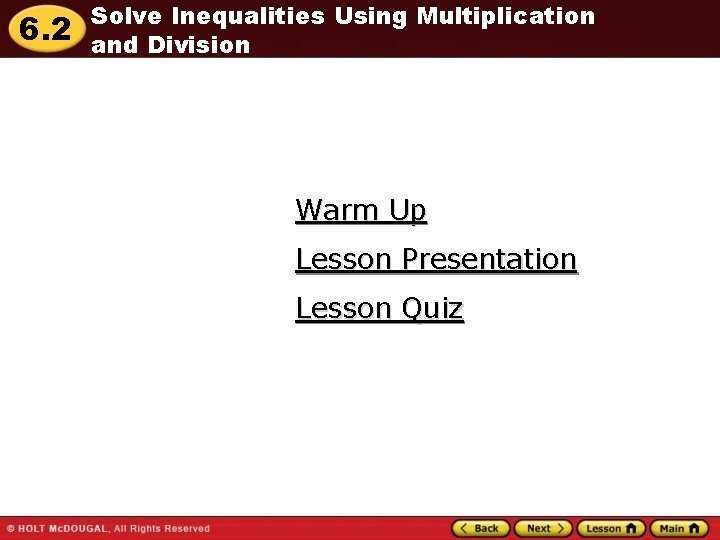6. 2 Solve Inequalities Using Multiplication and Division Warm Up Lesson Presentation Lesson Quiz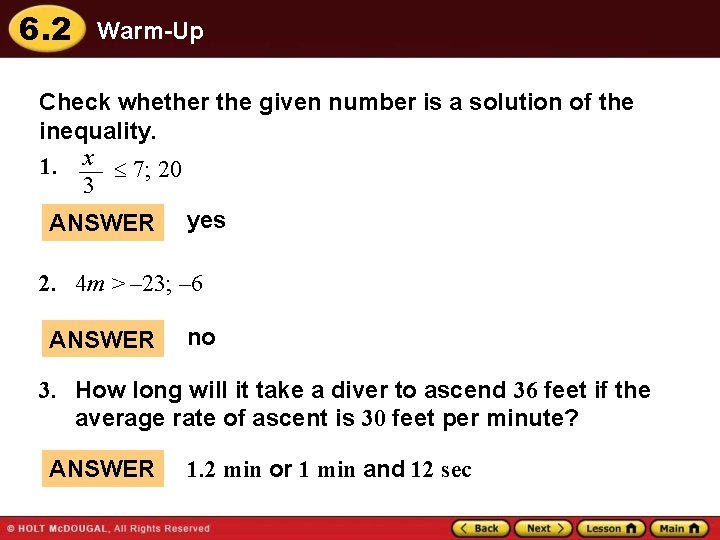6. 2 Warm-Up Check whether the given number is a solution of the inequality. 1. x 7; 20 3 ANSWER yes 2. 4 m > – 23; – 6 ANSWER no 3. How long will it take a diver to ascend 36 feet if the average rate of ascent is 30 feet per minute? ANSWER 1. 2 min or 1 min and 12 sec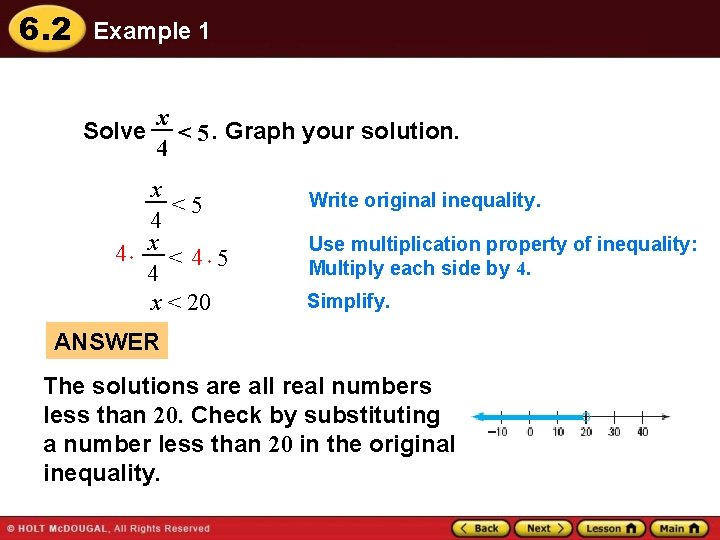6. 2 Example 1 Solve x < 5. Graph your solution. 4 x <5 4 4 x <4 5 4 x < 20 Write original inequality. Use multiplication property of inequality: Multiply each side by 4. Simplify. ANSWER The solutions are all real numbers less than 20. Check by substituting a number less than 20 in the original inequality.6. 2 Guided Practice Solve the inequality. Graph your solution. x 1. >8 3 ANSWER 2. x > 24 m – 2 8 ANSWER y 3. 2. 5 m – 16 – 4 ANSWER y – 10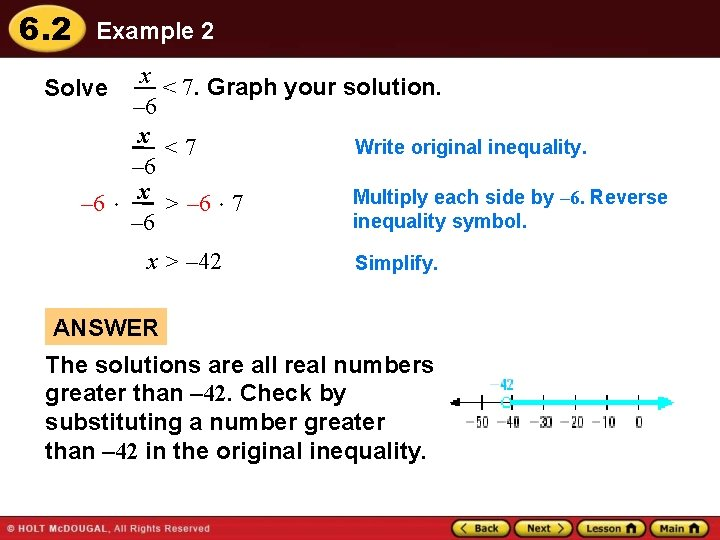6. 2 Example 2 x < 7. Graph your solution. Solve – 6 x Write original inequality. <7 – 6 Multiply each side by – 6. Reverse – 6 x > – 6 7 inequality symbol. – 6 x > – 42 Simplify. ANSWER The solutions are all real numbers greater than – 42. Check by substituting a number greater than – 42 in the original inequality.6. 2 Example 2 CHECK x – 6 <7 0 – 6 ? <7 0<7 Write original inequality. Substitute 0 for x. Solution checks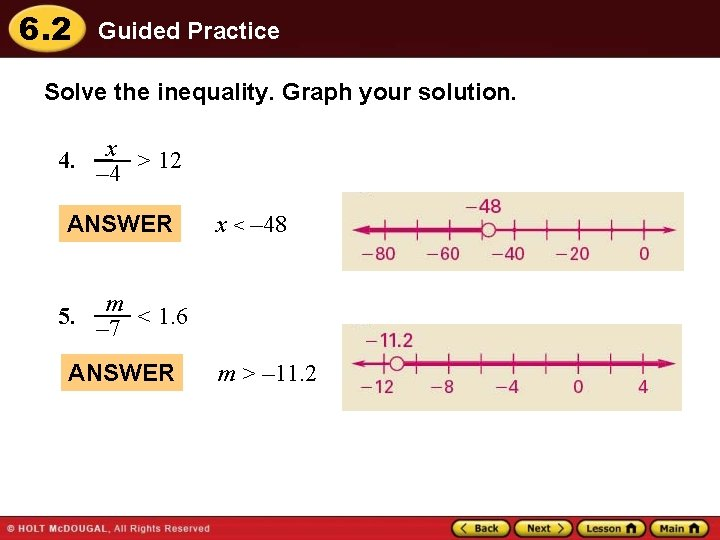6. 2 Guided Practice Solve the inequality. Graph your solution. x 4. > 12 – 4 ANSWER 5. x < – 48 m < 1. 6 – 7 ANSWER m > – 11. 2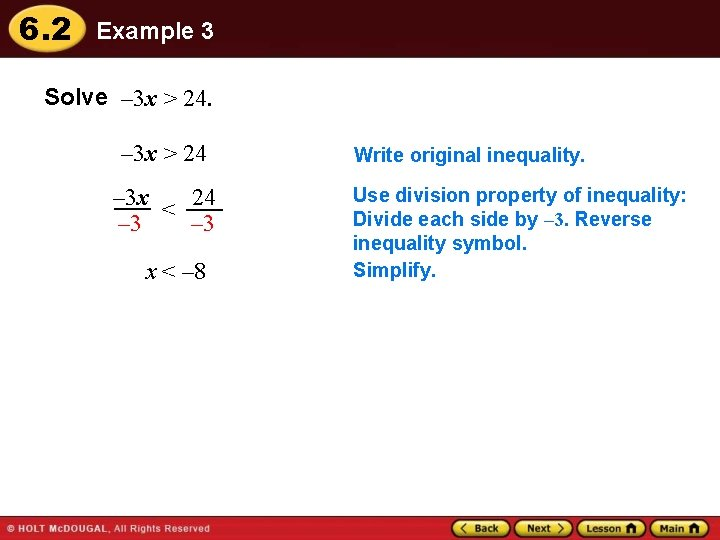6. 2 Example 3 Solve – 3 x > 24 – 3 x < 24 – 3 x < – 8 Write original inequality. Use division property of inequality: Divide each side by – 3. Reverse inequality symbol. Simplify.6. 2 Guided Practice Solve the inequality. Graph your solution. 6. 5 v 45 ANSWER v 9 7. – 6 n < 24 ANSWER n > – 4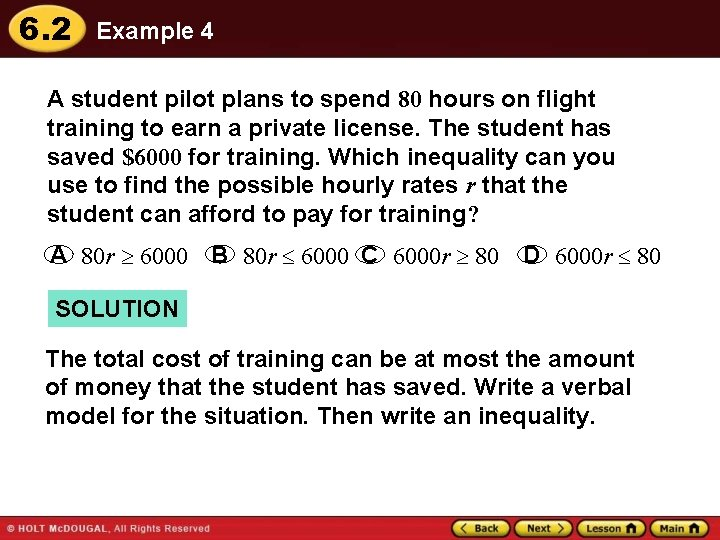6. 2 Example 4 A student pilot plans to spend 80 hours on flight training to earn a private license. The student has saved \$6000 for training. Which inequality can you use to find the possible hourly rates r that the student can afford to pay for training? A 80 r 6000 B 80 r 6000 C 6000 r 80 D 6000 r 80 SOLUTION The total cost of training can be at most the amount of money that the student has saved. Write a verbal model for the situation. Then write an inequality.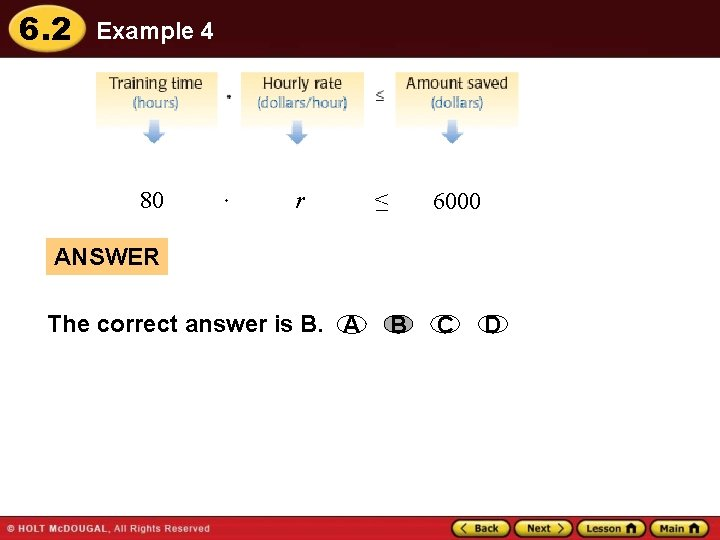6. 2 Example 4 80 r < – 6000 ANSWER The correct answer is B. A B C D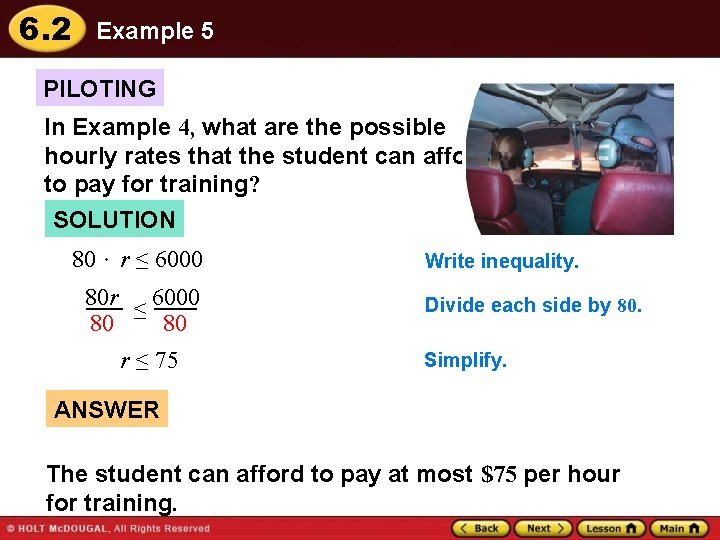6. 2 Example 5 PILOTING In Example 4, what are the possible hourly rates that the student can afford to pay for training? SOLUTION 80 r ≤ 6000 80 80 r ≤ 75 Write inequality. Divide each side by 80. Simplify. ANSWER The student can afford to pay at most \$75 per hour for training.6. 2 Guided Practice 8. WHAT IF? In Example 5, suppose the student plans to spend 90 hours on flight training and has saved \$6300. Write and solve an inequality to find the possible hourly rates that the student can afford to pay for training. ANSWER 90 r 6300, r \$70/h6. 2 Lesson Quiz Solve the inequality. Graph your solution. 1. – 72 < 8 p ANSWER 2. p > – 9 w > – 5 – – 6 ANSWER w 30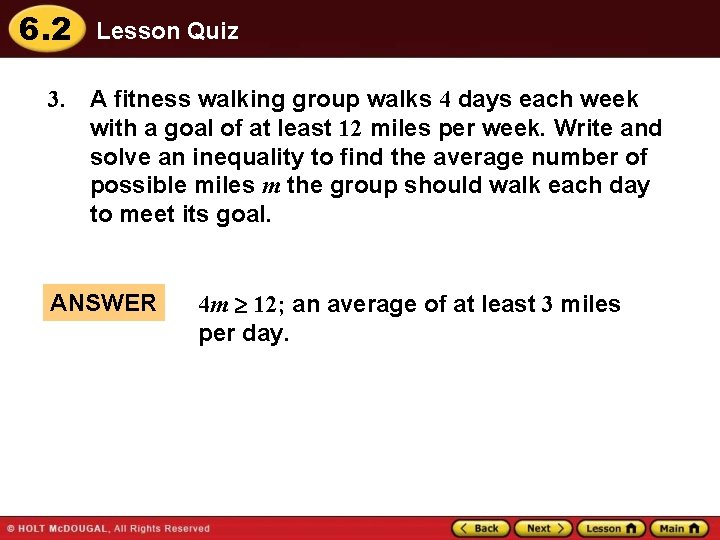6. 2 Lesson Quiz 3. A fitness walking group walks 4 days each week with a goal of at least 12 miles per week. Write and solve an inequality to find the average number of possible miles m the group should walk each day to meet its goal. ANSWER 4 m 12; an average of at least 3 miles per day.